### Today’s Puzzle:

These somewhat tricky level-5 puzzles are probably better suited for middle school and up than younger kids. Use logic on every step and you should be able to find its unique solution.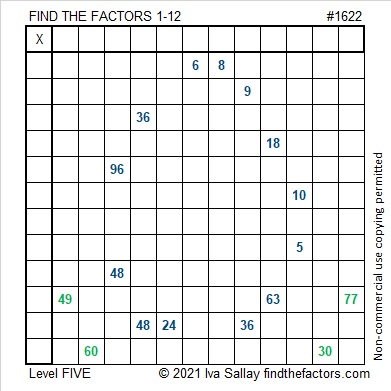Here are some Easter egg puzzles I saw on Twitter. Some are perfect for the littles and others are for older kids. Easter egg hunts can be fun for anyone of any age.

### Factors of 1622:

• 1622 is a composite number.
• Prime factorization: 1622 = 2 × 811.
• 1622 has no exponents greater than 1 in its prime factorization, so √1622 cannot be simplified.
• The exponents in the prime factorization are 1 and 1. Adding one to each exponent and multiplying we get (1 + 1)(1 + 1) = 2 × 2 = 4. Therefore 1622 has exactly 4 factors.
• The factors of 1622 are outlined with their factor pair partners in the graphic below.### More about the Number 1622:

1622 is the sum of four consecutive numbers:
409 + 410 + 411 + 412 = 1622.

# 1619 A Pink Egg Hidden in the Grass

### Today’s Puzzle:

Easter is less than two weeks away. This pink puzzle is the first of three level-5 Easter eggs hidden amongst some blades of grass for you to find and solve. The puzzle might be a little tricky, but use logic every step of the way, and you’ll be able to find the unique solution:### Factors of 1619:

• 1619 is a prime number.
• Prime factorization: 1619 is prime.
• 1619 has no exponents greater than 1 in its prime factorization, so √1619 cannot be simplified.
• The exponent in the prime factorization is 1. Adding one to that exponent we get (1 + 1) = 2. Therefore 1619 has exactly 2 factors.
• The factors of 1619 are outlined with their factor pair partners in the graphic below.

How do we know that 1619 is a prime number? If 1619 were not a prime number, then it would be divisible by at least one prime number less than or equal to √1619. Since 1619 cannot be divided evenly by 2, 3, 5, 7, 11, 13, 17, 19, 23, 29, 31, or 37, we know that 1619 is a prime number.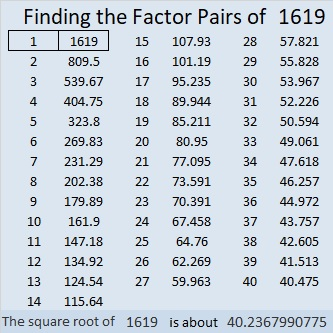### More about the Number 1619:

1619 is the sum of two consecutive numbers:
809 + 810 = 1619.

1619 is also the difference of two consecutive squares:
810² – 809² = 1619.

What do you think about that?

# 1609 Pot of Gold

### Today’s Puzzle:

They say at the end of the rainbow, there is a pot of gold that belongs to some leprechaun. Because this is a Find the Factors 1 to 14 puzzle, this pot of gold has some choice mathematical nuggets. For example, is 7 or 14 the common factor of 70 and 84? Don’t guess which one is the common factor for the puzzle. Use logic to eliminate one of those possibilities instead.

Likewise, both 6 and 9 are common factors of 18 and 54. And 4, 5, and 10 are all common factors of 20 and 40. Logic will narrow each possibility down to one possible factor!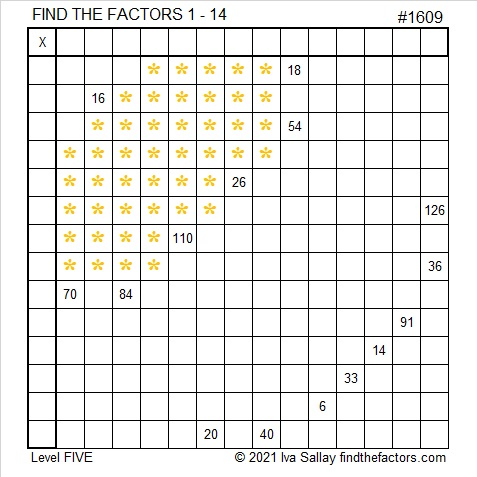Print the puzzles or type the solution in this excel file: 14 Factors 1604-1612.

I like that you also need to find the common factor of 126 and 36. I noticed a pattern with those clues. The pattern is limited to the multiplication facts given below, but I think it is still a pretty cool pattern.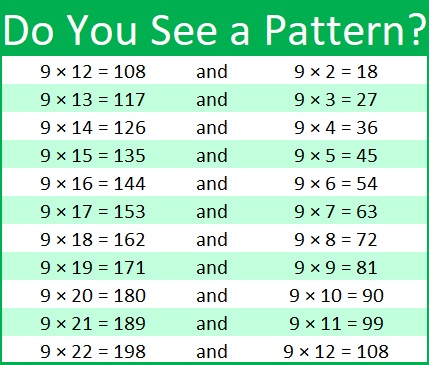Here’s the pattern I saw for 126 and 36:

• Since 9 is one of the factors, the sum of the digits of any of the products equals 9.
• 1 + 2 = 3. The sum of the first two numbers of the product in the first column equals the first part of the product in the second column.
• Obviously, both clues end in 6 so the last digit of their other factors will end with the same number, 4.

That should give you a good start in solving the puzzle!

### Factors of 1609:

• 1609 is a prime number.
• Prime factorization: 1609 is prime.
• 1609 has no exponents greater than 1 in its prime factorization, so √1609 cannot be simplified.
• The exponent in the prime factorization is 1. Adding one to that exponent we get (1 + 1) = 2. Therefore 1609 has exactly 2 factors.
• The factors of 1609 are outlined with their factor pair partners in the graphic below.

How do we know that 1609 is a prime number? If 1609 were not a prime number, then it would be divisible by at least one prime number less than or equal to √1609. Since 1609 cannot be divided evenly by 2, 3, 5, 7, 11, 13, 17, 19, 23, 29, 31, or 37, we know that 1609 is a prime number.### More about the Number 1609:

1609² = 2588881. That’s a perfect square, followed by four 8’s, followed by a perfect square. OEIS.org reports that 1609² is the smallest perfect square with four 8’s in a row.

1609 is the sum of two squares:
40² + 3² = 1609.

1609 is the hypotenuse of a Pythagorean triple:
240-1591-1609, calculated from 2(40)(3), 40² – 3², 40² + 3².

Here’s another way we know that 1609 is a prime number: Since its last two digits divided by 4 leave a remainder of 1, and 40² + 3² = 1609 with 40 and 3 having no common prime factors, 1609 will be prime unless it is divisible by a prime number Pythagorean triple hypotenuse less than or equal to √1609. Since 1609 is not divisible by 5, 13, 17, 29, or 37, we know that 1609 is a prime number.

# 1599 and Level 5

### Today’s Puzzle:

Write the numbers 1 to 10 in both the first column and the top row so that this level 5 puzzle will function like a multiplication table. Use logic with every step.### Factors of 1599:

• 1599 is a composite number.
• Prime factorization: 1599 = 3 × 13 × 41.
• 1599 has no exponents greater than 1 in its prime factorization, so √1599 cannot be simplified.
• The exponents in the prime factorization are 1, 1, and 1. Adding one to each exponent and multiplying we get (1 + 1)(1 + 1)(1 + 1) = 2 × 2 × 2 = 8. Therefore 1599 has exactly 8 factors.
• The factors of 1599 are outlined with their factor pair partners in the graphic below.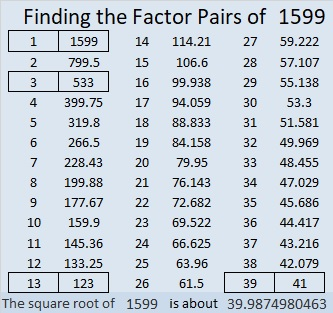### More about the Number 1599:

1599 is the hypotenuse of FOUR Pythagorean triples:
276-1575-1599, which is 3 times (92-525-533),
351-1560-1599, which is 39 times (9-40-41),
615-1476-1599, which is (5-12-13) times 123, and
924-1305-1599, which is 3 times (308-435-533).

1599 is the difference of two squares four different ways:
800² – 799² = 1599,
268² – 265² = 1599,
68² – 55² = 1599, and
40² – 1² = 1599.
Yes, we are just one number away from a perfect square!

# 1590 A Single Rose

### Today’s Puzzle:

A single rose can be an elegant expression of affection. This single rose is a level 5 puzzle. Can you find its factors?Here’s the same puzzle without any added color:### Two Factor Trees for 1590:

There are several possible factor trees for 1590. Here are two of them.### Factors of 1590:

• 1590 is a composite number.
• Prime factorization: 1590 = 2 × 3 × 5 × 53.
• 1590 has no exponents greater than 1 in its prime factorization, so √1590 cannot be simplified.
• The exponents in the prime factorization are 1, 1, 1, and 1. Adding one to each exponent and multiplying we get (1 + 1)(1 + 1)(1 + 1)(1 + 1) = 2 × 2 × 2 × 2 = 16. Therefore 1590 has exactly 16 factors.
• The factors of 1590 are outlined with their factor pair partners in the graphic below.### More about the Number 1590:

1590 is the hypotenuse of FOUR Pythagorean triples:
138-1584-1590, which is 6 times (23-264-265),
576-1482-1590, which is 6 times (96-247-265)
840-1350-1590, which is 30 times (28-45-53),
954-1272-1590, which is (3-4-5) times 318.

# 1581 One Teardrop among Millions

### Today’s Puzzle:

Earlier this week the United States surpassed 400,000 deaths from the novel coronavirus. Over 2,000,000 people have died from the virus worldwide. Most of these people died in isolation away from loved ones. Most of the millions of tears shed have also been in isolation. New and more contagious strains of the virus have arisen in various parts of the world threatening to make the death toll worse and the number of tears to grow exponentially. Each of us can and must do our part to flatten the curve and thus restrict the number of deaths and the number of tears.### Factors of 1581:

• 1581 is a composite number.
• Prime factorization: 1581 = 3 × 17 × 31.
• 1581 has no exponents greater than 1 in its prime factorization, so √1581 cannot be simplified.
• The exponents in the prime factorization are 1, 1, and 1. Adding one to each exponent and multiplying we get (1 + 1)(1 + 1)(1 + 1) = 2 × 2 × 2 = 8. Therefore 1581 has exactly 8 factors.
• The factors of 1581 are outlined with their factor pair partners in the graphic below.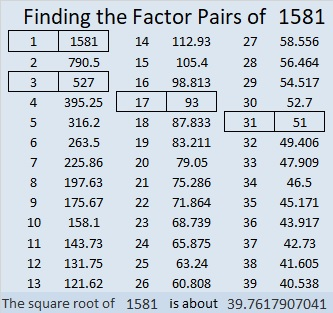### Another Fact about the Number 1581:

1581 is the hypotenuse of a Pythagorean triple:
744-1395-1581, which is (8-15-17) times 93.

# 1563 The Holly Wreath

### Today’s puzzle:

A holly wreath is yet another symbol that connects Christmas with Easter. It symbolizes eternity in its color and shape. It bears white flowers, red berries, and thorns reminding us of purity, blood, and a crown of thorns.

You might find some of the clues in this level 5 puzzle to be like thorns, but don’t give up. Use logic and perseverance and you will be able to find its unique solution.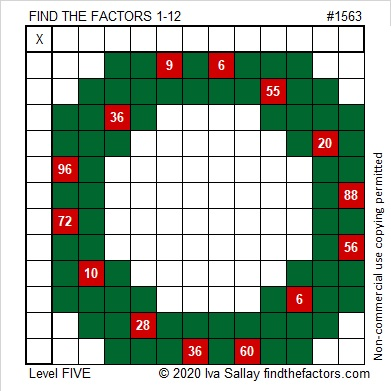Here’s the same puzzle without all the added color: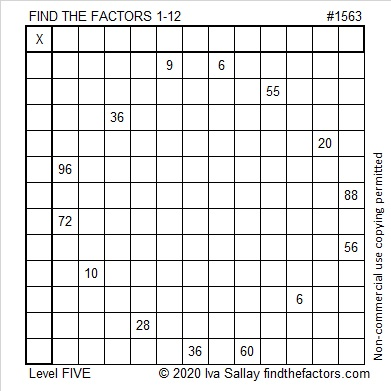### Factors of 1563:

• 1563 is a composite number.
• Prime factorization: 1563 = 3 × 521.
• 1563 has no exponents greater than 1 in its prime factorization, so √1563 cannot be simplified.
• The exponents in the prime factorization are 1 and 1. Adding one to each exponent and multiplying we get (1 + 1)(1 + 1) = 2 × 2 = 4. Therefore 1563 has exactly 4 factors.
• The factors of 1563 are outlined with their factor pair partners in the graphic below.### Another Fact about the Number 1563:

1563 is the hypotenuse of a Pythagorean triple:
837-1320-1563, which is 3 times (279-440-521).

# 1552 Look for Clues around the Corner

### Today’s Puzzle:

This puzzle has four sets of clues that turn the corner. You will need to look around those corners to solve it. Use logic and have fun!### Factors of 1552:

• 1552 is a composite number.
• Prime factorization: 1552 = 2 × 2 × 2 × 2 × 97, which can be written 1552 = 2⁴ × 97.
• 1552 has at least one exponent greater than 1 in its prime factorization so √1552 can be simplified. Taking the factor pair from the factor pair table below with the largest square number factor, we get √1552 = (√16)(√97) = 4√97.
• The exponents in the prime factorization are 4 and 1. Adding one to each exponent and multiplying we get (4 + 1)(1 + 1) = 5 × 2 = 10. Therefore 1552 has exactly 10 factors.
• The factors of 1552 are outlined with their factor pair partners in the graphic below.### More about the Number 1552:

1552 is the sum of two squares:
36² + 16²  = 1552

1552 is the hypotenuse of a Pythagorean triple:
1040-1152-1552, calculated from 36² – 16², 2(36)(16), 36² + 16².
It is also 16 times (65-72-97).

OEIS.org looked around the corner at the two numbers preceding 1552 to find something special about that number: The sum of its prime factors equals the sum of the prime factors of those previous two numbers! That’s a cool enough fact that I decided to make this graphic:# 1530 Jack-o’-lantern

### Today’s Puzzle:

Here’s a Jack-O’-Lantern Puzzle for you to enjoy. It’s a Level 5 puzzle so it might be more of a trick than a treat. Remember to use logic every step of the way instead of guessing and checking.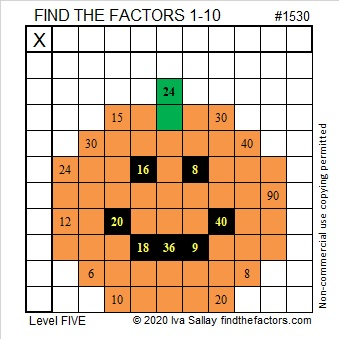Here’s the same puzzle without any added color:### Factors of 1530:

15 is half of 30, so 1530 is divisible by 6 just like all these numbers are divisible by 6: 12, 24, 36, 48, 510, 612, 714, 816, 918, 1020, 1122, 1224, 1326, 1428, and so forth.

• 1530 is a composite number.
• Prime factorization: 1530 = 2 × 3 × 3 × 5 × 17, which can be written 1530 = 2 × 3² × 5 × 17.
• 1530 has at least one exponent greater than 1 in its prime factorization so √1530 can be simplified. Taking the factor pair from the factor pair table below with the largest square number factor, we get √1530 = (√9)(√170) = 3√170.
• The exponents in the prime factorization are 1, 2, 1, and 1. Adding one to each exponent and multiplying we get (1 + 1)(2 + 1)(1 + 1)(1 + 1) = 2 × 3 × 2 × 2 = 24. Therefore 1530 has exactly 24 factors.
• The factors of 1530 are outlined with their factor pair partners in the graphic below.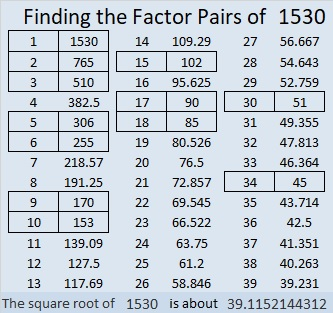### More about the Number 1530:

51 × 30 = 1530. Did you notice that the same digits appear on both sides of the equal sign and only +, -, ×, ÷, (), or exponents were used to make a true statement? 1530 is only the 25th number that can make that claim, so we call it the 25th Friedman number.

There are MANY possible factor trees for 1530, but let’s celebrate that it is also a Friedman number with this one: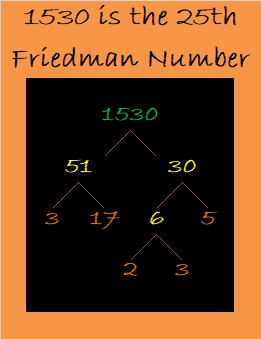1530 is the hypotenuse of FOUR Pythagorean triples:
234-1512-1530, which is 18 times (13-84-85),
648-1386-1530, which is 18 times (36-77-85),
720-1350-1530, which is (8-15-17) times 90, and
918-1224-1530, which is (3-4-5) times 306.

# 1517 and Level 5

### Today’s Puzzle:

Which common factor of 72 and 36 is needed to solve this puzzle? Is it 6, 9, or 12? There is an easier place to begin this level 5 puzzle. Don’t guess and check. Use logic to know which factors you should use.  You can figure it out!### Factors of 1517:

• 1517 is a composite number.
• Prime factorization: 1517 = 37 × 41.
• 1517 has no exponents greater than 1 in its prime factorization, so √1517 cannot be simplified.
• The exponents in the prime factorization are 1, and 1. Adding one to each exponent and multiplying we get (1 + 1)(1 + 1) = 2 × 2 = 4. Therefore 1517 has exactly 4 factors.
• The factors of 1517 are outlined with their factor pair partners in the graphic below.### More Facts about the Number 1517:

1517 is the difference of two squares in two different ways:
759² – 758² = 1517,
39² – 2² = 1517.

1517 is also the sum of two squares in two different ways:
34² + 19² = 1517,
29² + 26² = 1517.

1517 is the hypotenuse of FOUR Pythagorean triples:
165-1508-1517, calculated from 29² – 26², 2(29)(26), 29² + 26²,
333-1480-1517, which is 37 times (9-40-41),
492-1435-1517, which is (12-35-37) times 41,
795-1292-1517, calculated from 34² – 19², 2(34)(19), 34² + 19².